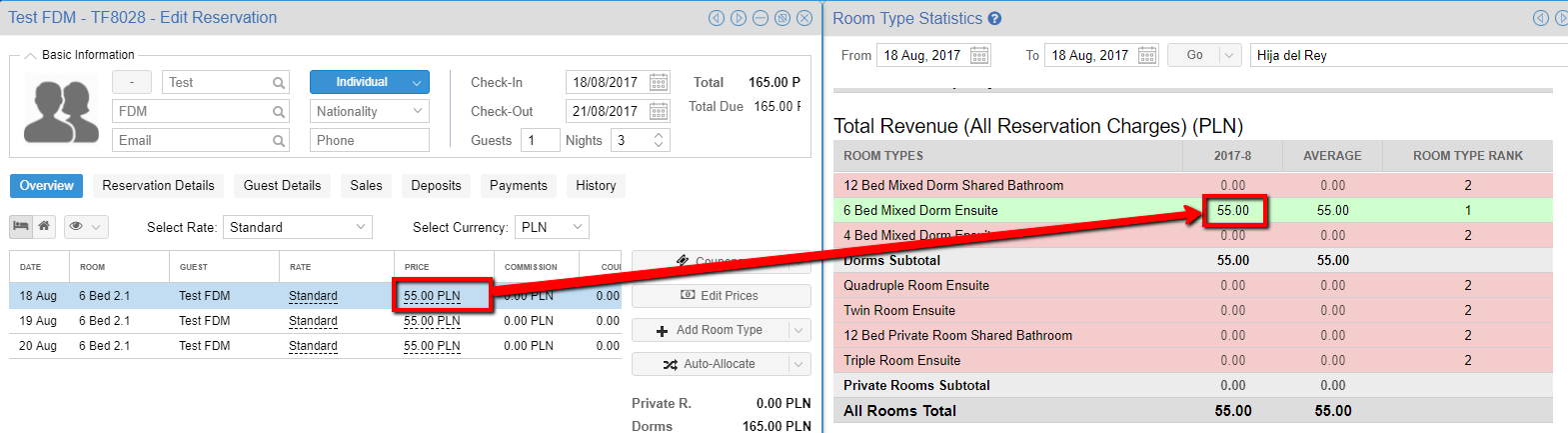In Room Type Statistics Report you can find the following information:

1) % Occupancy

The occupancy, in this case, is counted per bed:

Single bed = 1

Double bed = 1

Double bank = 2

The applied formula is: room type total number of beds / occupied beds

The system divides the total number of bed in each room type by occupied beds.

2) Total Revenue (only accommodation)

• Coupons are discounted
• Commissions are NOT discounted
• City tax is not included

The system sums the booking prices from the selected period. E.g. if we have a booking for the dates 18-20.08 and we want to generate the report for 18.08, it will show only the 18.08 price.3) Average Daily Revenue

In this case, the applied formula is: total revenue (all room total from the point 2) / number of days

4) Average Daily Rate

In this case, the applied formula is: room type total revenue (point 2) / room type occupied beds

We have 30 beds in the hostel. 15 of them are occupied. Now if we have e.g 10-day total bookings revenue 35600, we have to divide this number by the sum of each day occupied beds. The calculation would be 35600 / 150 (10 days x 15 beds).

5) RevPAB (Dorms) & RevPAR (Private Rooms)

RevPAR (revenue per available room) is a performance metric in the hotel industry. It allows assessing the property's ability to fill its available rooms at an average rate. It can be calculated by Occupancy x Average Daily Rate = RevPAR

RevPAB (revenue per available bed) is the analogical metrics but measured per bed not per room.

6) Revenue Per Square Meter

In this case, the applied formula is: total revenue/room surface sum

7) Total Number of Bookings

The total number of bookings which stays or arrives during the selected period.

8) Total Number of BedNights

Total of occupied beds.

Single = 1

Double = 2

Double Bunk = 2

9) Average Number of Guests Per Night

In this case, the applied formula is: total number of BedNights / number of days

10) Average Number of BedNights per Reservation

In this case, the applied formula is: total of BedNights / number of bookings